# The Black–Litterman model explained

## Abstract

Active portfolio management is about leveraging information. The Black and Litterman Global Portfolio Optimisation Model (BL) sets information processing in a Bayesian analytic framework. In this framework, the portfolio manager needs only produce views and the model translates the views into security return forecasts. As a portfolio construction tool, the BL model is appealing both in theory and in practice. Although there has been no shortage of literature exploring it, the model still appears somehow mysterious, and suffers from practical issues. This article is dedicated to enabling a better understanding of this model, and features:

• an economic interpretation;

• a clarification of the model's assumptions and formulation;

• implementation guidance;

• a dimension-reduction technique to enable large portfolio applications; and

• a full proof of the main result in the Appendix.

We also provide a checklist of other practical issues that we aim to address in our forthcoming articles.

This is a preview of subscription content, access via your institution.

1. Views are often also expressed in terms of fundamental or macroeconomic factors. In a forthcoming article, we will develop a technique for factor-based allocation.

2. In this article, we use upper-case R to stand for total return and lower-case r for excess return, that is, r=Rr f , where r f is the risk-free rate.

3. In this article, we use ‘’ to denote a random variable;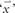to denote a vector; and a bold symbol (‘X’) to denote a matrix. The dimension(s) of vector and matrix will be clarified on its first appearance. For example,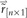stands for the n by 1 return vector with random entries.

4. In this article,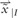is used to denote our perception of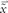after examining the information I. As in the Bayesian framework updated perceptions are still considered random, we need such notation to distinguish them from mean (point) estimates5. As is usual, we use ‘◯’ to denote an estimate for x. Note in the traditional framework, estimates are deterministic; whereas in the Bayesian framework estimates are still random, but with updated uncertainty.

6. See Black and Litterman (1992) for a description and critiques of these methods.

7. Unless otherwise specified, in this article all returns refer to excess returns.

8. Normally, analysts/PMs form their views according to information arrivals. In the remainder of the article, we use view and information interchangeably.

9. In the Bayesian framework, views should also be treated as random, as there are always forecast errors.

10. As we develop more insights in our forthcoming articles, we will see that τ is a subjective parameter and that such restriction does not exist.

11. This assumption comes as a convention of the classical linear regression model. This provides some simplicity. However, as far as the model is concerned, this independence restriction is not necessary.

12. Careful readers may have noted that the relationship (3) can be re-arranged and considered as a linear system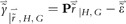for fitting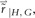withand P as data. It seems thatcan be estimated by the generalised least square (GLS) estimatorThere are, however, two reasons why the following BL solution is more practical: (1) In practice, it is often the case that we only have k (⩽n) views. As such, the matrix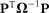is singular and therefore the GLS estimation is not attainable. (2) Treatingand P as data is too optimistic – these are often just analysts’ opinions. The strong associated uncertainty makes the Bayesian technique a more natural fit.

## References

• Black, F. and Litterman, R. (1992) Global portfolio optimisation. Financial Analysts Journal 48 (5): 28–43.

• Fama, E.F. (1970) Efficient capital markets: A review of theory and empirical work. Journal of Finance 25 (2): 383–417.

• Hamilton, J. (1994) Time Series Analysis. Princeton, NJ: Princeton University Press.

• Idzorek, T.M. (2004) A Step-by-Step Guide to the Black–Litterman Model. Zephyr Working Document 2004.

• Jones, R., Lim, T. and Zangari, P.J. (2007) The Black–Litterman model for structured equity portfolios. Journal of Portfolio Management 33 (2): 24–33.

• Martellini, L. and Ziemann, V. (2007) Extending Black–Litterman analysis beyond the mean-variance framework. Working Document, EDHEC.

• Meucci, A. (2005) Beyond Black–Litterman: Views on non-normal market. Available at SSRN: http://ssrn.com/abstract=848407.

• Satchell, S. and Scowcroft, A. (2000) A demystification of the Black–Litterman model: Managing quantitative and traditional portfolio construction. Journal of Asset Management 1 (2): 138–150.

• Zhou, G. (2008) An extension of the Black–Litterman model: Letting the data speak. Working Document, Washington University in St Louis.

## Acknowledgements

I thank Bob Litterman, Ronny Feiereisen, Alan Hofmeyr, Wuan Luo, Amit Manwani, Attilio Meucci, Mayank Mishra, Stephen Vandermark and numerous European and US quant fund managers for helpful discussions. All mistakes are mine.

Authors

## Appendix

### Proof

The full proof of Theorem 1 is given below.

### Theorem 1 (Posterior Return Estimates)

• The posterior return vector is normally distributed, that is,where the updated mean vector is: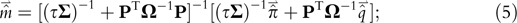and the updated variance-covariance matrix is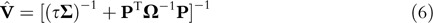### Proof

• We first deal with a general case and then apply it into the BL context. In general, assume the following linear relationship: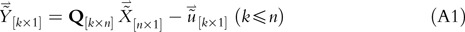where Q is a known/observable matrix;is an observable vector;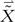is unobservable and needs to be estimated; and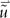is the error vector.

Also, assume the following Gaussian distributions for regression errors and the prior: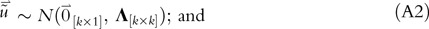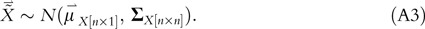We therefore have conditional on a realisation of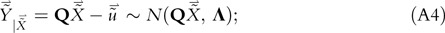In terms of probability density function (pdf), distributions (A3) and (A4) can be equivalently written as: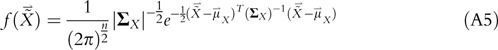where ∣·∣ gives the determinant of the matrix it applies to; and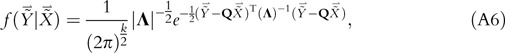respectively.

We try to imply the probability distribution of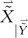from the joint pdf: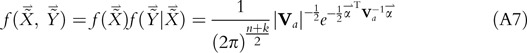where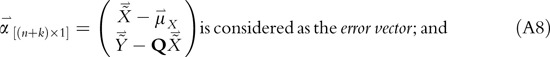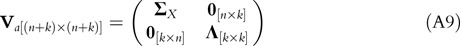We hope, based on the Bayes’ Rule, that it is possible to express (A7) in terms of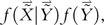as we are interested in the posterior estimation of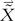given. This translates into a need to replace the dependence of the mean estimates of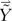on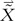(As in (A4)) with a dependence of the mean estimates of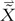on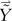. In other words, through some transformation, we need to get rid of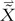from the lower part of the error vector in (A8), but allow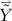to enter the upper part. To this end, we construct the following matrix (Hamilton, 1994, Ch.12):where note ∣A∣=1.

Using A as the transform matrix, we define:where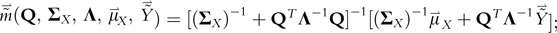and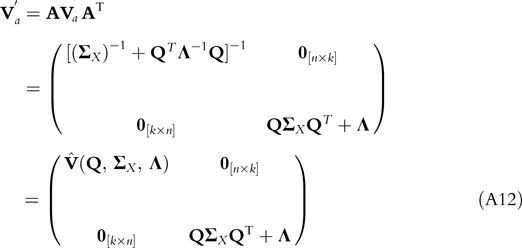where (Q, Σ x , Λ)=[(Σ x )−1+QTΛ−1Q]−1.

Therefore, (A7) can be rearranged, noting ∣A−1∣=∣A∣=1, as follows: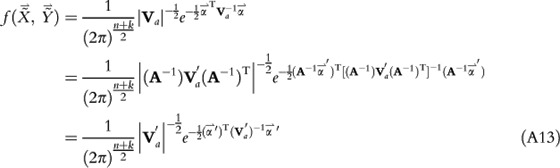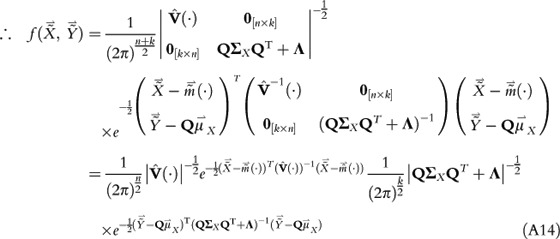where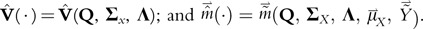From (A1), (A2) and (A3), we have the unconditional distribution: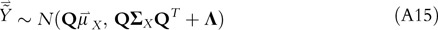With (A15), it is easy to imply from (A14) the following: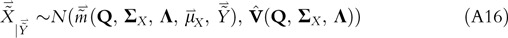To assessin (A16), we use our best knowledge regarding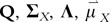and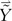.

Recall that in the model setting our best knowledge based on the public information G leads to the following prior belief: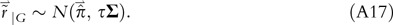After examining the private information H, we form the following updated views:where P is the view structure;is the view forecast vector; and we assume.

Therefore, conditional on a realisation of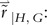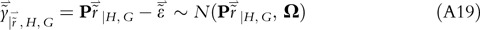Substituting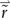for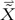and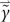for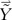into (A16), we reach:Finally, using our eventual conviction about the mean of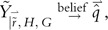we reach the following posterior belief: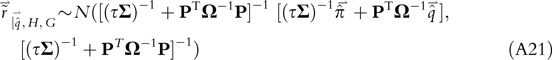This completes the proof. □

## Rights and permissions

Reprints and Permissions

Cheung, W. The Black–Litterman model explained. J Asset Manag 11, 229–243 (2010). https://doi.org/10.1057/jam.2009.28

• Revised:

• Published:

• Issue Date:

• DOI: https://doi.org/10.1057/jam.2009.28

### Keywords

• portfolio construction
• Bayes’ Rule
• view blending and shrinkage
• semi-strong market efficiency
• optimisation
• robustness